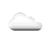## Learning Python: How to draw a scatter plot using plotly

In my earlier plot How to use FOR loop, I used a look to generate camera positions for a Panda3D view. In this post, I will show how to use plotly to visualize your data. To visualize camera positions generated by the FOR loop, I just need to show points at locations for all the angular steps generated. A good way to show these camera position is use scatter plot. In scatter plot, you will provide x & y coordinates of points that you want to show. Following code sample shows how to use plotly to draw Scatter plot.```from plotly import express as px
from math import pi,sin,cos

class CameraPosition:
def __init__(self, x, y, z=0):
self.x = x
self.y = y
self.z = z

angle_step = 6.0
camera_positions = []
for step in range(1,60):
angle_degrees = step * angle_step
angle_radians = angle_degrees * (pi / 180.0)
camera_positions.append(CameraPosition(x_new,y_new))

chart = px.scatter(x=[pos.x for pos in camera_positions],
y=[pos.y for pos in camera_positions], width=800, height=800)
chart.update_layout(title="Camera Positions")
chart.show()
```

The fist line of the code imports express module from plotly package. To install plotly package, run the following command on your machine.

pip install plotly

In subsequent posts, I will show to draw other types of visualizations depending on type of data and purpose of visualization.

## Weather

#### 15.9 °C / 60.6 °FClouds